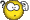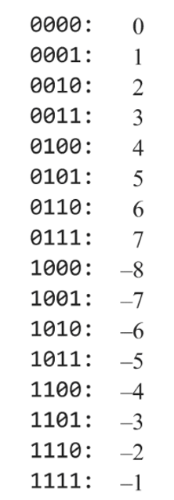# ConfusionClassicListThreaded7 messagesOpen this post in threaded view
|

## Confusion

 Hi. I am creating a visual diagram of the Hack ALU. Ive gotter pretty far. Im lost though when it comes to the output ng. Im not sure just exactly what a lesser than zero is. How do I connect 16 output and make a lesser than zero. Any suggestions?Open this post in threaded view
|

## Re: Confusion

 Administrator Let's say that I have the following 16-bit values: 0100010101001010 1110010100111101 0000010111101011 1111110111011100 1000000000000011 0000000000000000 1111111111111111 1111110000010111 0000000111101011 0111111111111111 Which of them are negative (strictly less than zero)? How did you decide which are negative and which are not?
Open this post in threaded view
|

## Re: Confusion

 In reply to this post by Dan1984mor2017 Look at how negative numbers are stored in 2's complement.  there is a bit that tells you if the number is < 0. hopefully that's all the hint you need....
Open this post in threaded view
|

## Re: Confusion

 In reply to this post by WBahn I think based on two's complement that it depends on the bit six like 16. So if their was only two bit the first two 00, 01 would be positive and the other would be negative. I suppose it is just like flipping the values. 10 would be negetive two. And 11 would be negetive one.
Open this post in threaded view
|

## Re: Confusion

 Thanks for the hint. I understand. I'm going to try to make a cup that will divide amongs the bits. Seems a little challenging. For 16 almost seems impossible. Especially being one output. Maybe I can just divide it. Im am going to start there.
 I have reproduced below a portion of Figure 2-1 from Chapter 2, Section 2.4 of The Elements of Computing Systems where the representation of signed numbers using the 2's complement method is explained.  This chart is for 4-bit binary numbers.Note that this list includes every possible signed 4-bit number.  Examine the binary representation carefully.  What do you notice about the most significant bit (the left-most bits) that relates to whether the number represented is negative or non-negative? Create a similar table for 5-bit signed integers using 2's complement representation.  Is the same fact still true? What about for 8-bit numbers? Still true? Is it also true for 16-bit signed numbers represented in 2's complement? This is the direction that WBahn is trying to direct you.  If you're still unsure, get a copy of the book and look at the next paragraphs after the figure above where the authors explicitly provide the answer. Using the property that you have discovered about 2's complement representation, it should be relatively straightforward to figure out how to set the proper value of the ng flag bit based on the current output of the ALU.